# Correlation and Regression

## Simple Linear Regression

Frequently, it is of interest to investigate the relationship between two variables where one variable, the predictor/explanatory variable (X), is thought of as driving/explaining the second variable, the response (Y). Both of these variables are assumed to be quantitative. Other names these variables may have are: dependent variable (Y) and independent variable (X).

### Steps in such investigation

1. Plot the data. In many cases the plot can tell us visually whether there seems to be a relationship: if there is some correlation, do the variables increase or decrease together?, does one decrease when the other increases? Also, is a straight line a suitable model to describe the relationship between the two variables, and so on. If we want to go beyond this qualitative level of analysis then simple linear regression is often a useful tool. This involves fitting a straight line through our data and investigating the properties of the fitted line. It is conventional to plot the Y- response variable on the vertical axis and the independent variable X on the horizontal axis.
2. Plot the line of best fit. If the the plot suggests a linear relationship, we proceed to quantify the relationship between the two variables by fitting a regression line through the data points. This regression line can be defined as:

Y =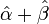$\hat\alpha + \hat\beta$X + ResidualAn example of fitting a quadratic function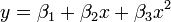$y=\beta_1+\beta_2x+\beta_3x^2\,$ (in blue) through a set of data points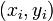$(x_i, y_i)$ (in red). In linear least squares the function need not be linear in the argument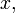$x,$ but only in the parameters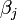$\beta_j$ that are determined to give the best fit.

(where a is the intercept, β is the slope of the line and the residual is the part that cannot be accounted for by the model) It is clear that all the points do not lie exactly on a straight line. The line fitted is by the least squares criterion (this the criterion which is almost invariably used). Using regression we can also fit many other types of models including those where we have more than one independent variable. The numbers a and β can be calculated as follows: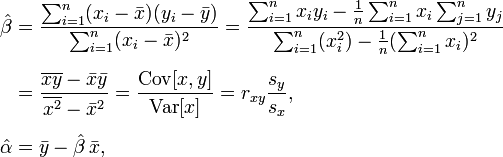\begin{align} \hat\beta & = \frac{ \sum_{i=1}^{n} (x_{i}-\bar{x})(y_{i}-\bar{y}) }{ \sum_{i=1}^{n} (x_{i}-\bar{x})^2 } = \frac{ \sum_{i=1}^{n}{x_{i}y_{i}} - \frac1n \sum_{i=1}^{n}{x_{i}}\sum_{j=1}^{n}{y_{j}}}{ \sum_{i=1}^{n}({x_{i}^2}) - \frac1n (\sum_{i=1}^{n}{x_{i}})^2 } \\[6pt] & = \frac{ \overline{xy} - \bar{x}\bar{y} }{ \overline{x^2} - \bar{x}^2 } = \frac{ \operatorname{Cov}[x,y] }{ \operatorname{Var}[x] } = r_{xy} \frac{s_y}{s_x}, \\[6pt] \hat\alpha & = \bar{y} - \hat\beta\,\bar{x}, \end{align}

where rxy is the sample correlation coefficient between x and y, sx is the standard deviation of x, and sy is correspondingly the standard deviation of y. A horizontal bar on top of a variable indicates the sample average of that variable.

Don't be intimidated by the above calculations! Any scientific calculator can calculate the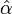$\hat\alpha$ and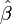$\hat\beta$ after inputting a series of paired values (x,y) and selecting linear regression. It will also calculate the r2, of which the square root is the Pearson Correlation Coefficient (or Product Moment correlation coefficient), which is used to quantify the nature of the linear relationship and thus used in Correlation Analysis.

## Correlation Analysis

Sometimes we do not have a clear predictor and a clear response variable., thus we may be interested in quantifying the relationship between a pair of variables. The regression of X on Y does not give the same regression line as the regression of Y on X. This is because regression analysis presupposes a directional relationship, i.e. X is thought of as influencing Y and not vice versa. Despite this, the r2 value obtained from both regressions will be the same. It is a measure of the strength of the linear relationship between X and Y, irrespective of which is considered to influence the other. The square root of r2 turns out to be exactly the same as a measure called the correlation coefficient (aka Pearson's correlation coefficient, Product Moment correlation coefficient) which was proposed to measure the strength of linear relationships between normally distributed random variables.

The correlation coefficient is just the square root of r2 but has a sign attached: it will be positive if X and Y increase and decrease together and negative if one increases while the other decreases.

• The correlation coefficient varies from -1 to + 1: it is -1 or + 1 if all the points lie in a straight line and zero if there is completely random scatter.
• Sign → direction (directly/inversely associated)
• Size (absolute value) → how close the points are clustered around a line (correlation)
• It is also crucial to remember that correlation does not imply causation (but merely association!).